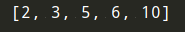# 《算法图解》学习笔记（二）：选择排序（附代码）

python学习之路-从入门到精通到大师文章目录[python学习之路-从入门到精通到大师](https://blog.csdn.net/TeFuirnever/article/details/90017382)一、内存的工作原理二、数组和链表1）链表2）数组3）术语4）在中间插入5）删除三、选择排序四、总结参考文章学习两种最基本的数据结构——数组和链表，它们无处不在。一、内存的工作原理假设你去看...python学习之路 - 从入门到精通到大师fe0ffeeb是一个内存单元的地址，就相当于是机器给你的那个小票。• 你额外请求的位置可能根本用不上，这将浪费内存。你没有使用，别人也用不了。
• 待办事项超过10个后，你还得转移。

1）链表2）数组3）术语4）在中间插入5）删除PS：

• 不同：链表是 链式 的存储结构；数组是 顺序 的存储结构。
• 相同：两种结构均可实现数据的顺序存储，构造出来的模型呈 线性结构。• 电话簿中的人名
• 旅行日期
• 电子邮件（从新到旧）

python版本代码如下：

`#查找数组中的最小值def findSmallest(arr):  #存储最小值  smallest = arr  #存储最小值的索引  smallest_index = 0  for i in range(1, len(arr)): if arr[i] < smallest:smallest_index = ismallest = arr[i]  return smallest_index#排序数组def selectionSort(arr):  newArr = []  for i in range(len(arr)):#查找数组中最小的元素并将其添加到新数组中smallest = findSmallest(arr)newArr.append(arr.pop(smallest))  return newArrprint(selectionSort([5, 3, 6, 2, 10]))`c 版本代码如下：

`#include <iostream>#include <vector>using std::cout;using std::endl;// Finds the smallest value in an arraytemplate <typename T>int find_smallest(const std::vector<T>& arr) { // stores smallest value T smallest = arr; // stores index of the smallest value int smallest_index = 0; for (int i = 0; i < arr.size(); i  ) {  if (arr[i] < smallest) {smallest = arr[i];smallest_index = i;  } } return smallest_index;}template <typename T>std::vector<T> selection_sort(std::vector<T> arr) { std::vector<T> sorted; while(!arr.empty()) {  // find smallest element and add it to sorted array  int smallest_index = find_smallest(arr);  sorted.push_back(arr[smallest_index]);  // remove smallest element from non-sorted array  arr.erase(arr.begin()smallest_index); } return sorted;}int main() { std::vector<float> arr = {1.2, 1.0, 3, 0, -1, 0.5, 100, -99}; std::vector<float> sorted = selection_sort(arr);  cout << "Sorted array: "; for (float num : sorted) {  cout << num << " "; } cout << endl;}`

• 计算机内存犹如一大堆抽屉。
• 需要存储多个元素时，可使用数组或链表。
• 数组的元素都在一起。
• 链表的元素是分开的，其中每个元素都存储了下一个元素的地址。
• 数组的读取速度很快。
• 链表的插入和删除速度很快。
• 在同一个数组中，所有元素的类型都必须相同（都为int、double等）。